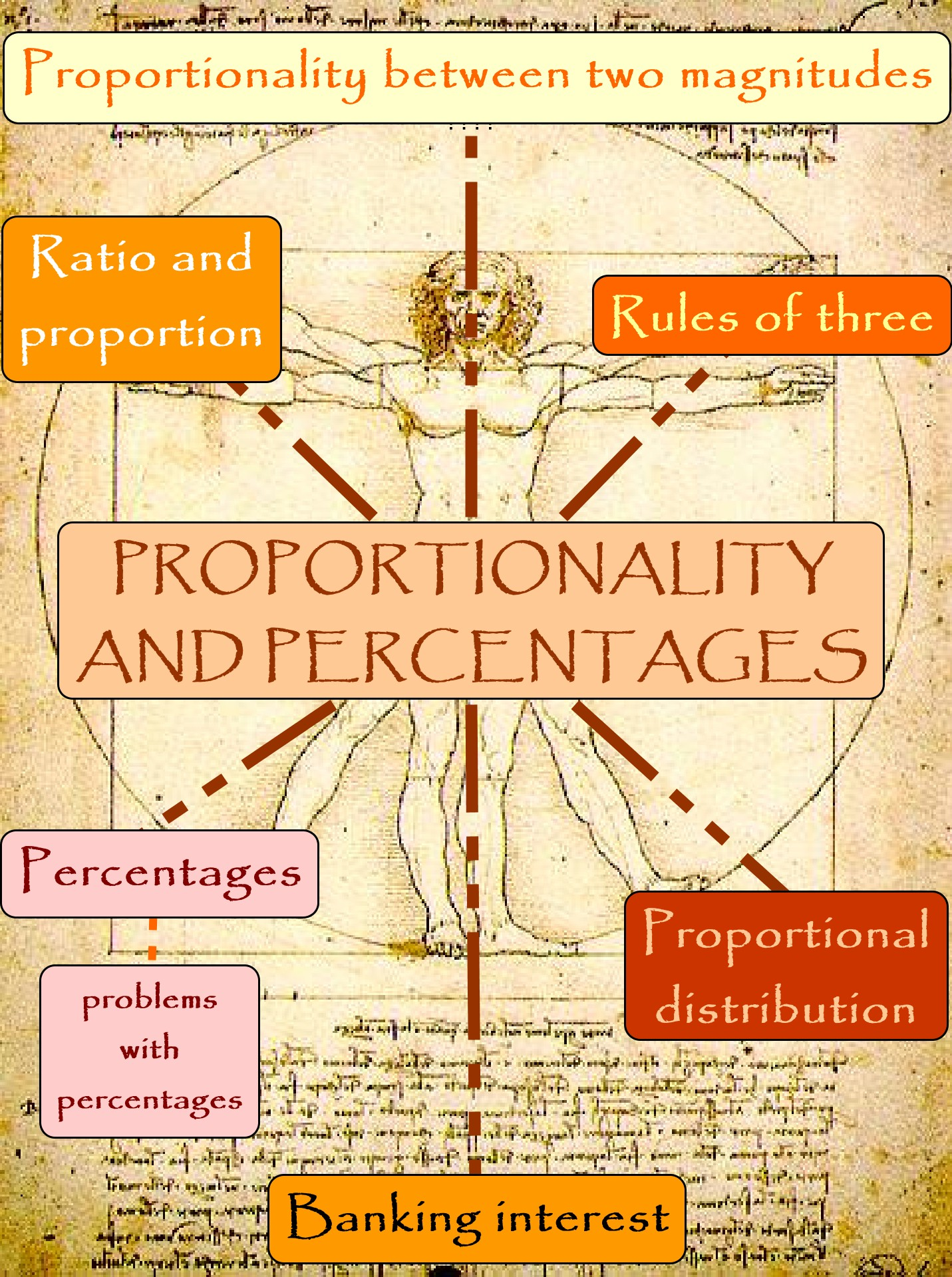All the community
search

# Proportionality and percentages

## Descripciónratio is a comparison of two numbers. We generally separate the two numbers in the ratio with a colon (:) or write it as a fraction. A proportion is one equality with a ratio on both sides.
percentage is a ratio of a number to 100. A percentage is expressed using the symbol %. A percentage is also equivalent to a fraction with denominator 100.
See the full teaching sequence

• Actions more_vert